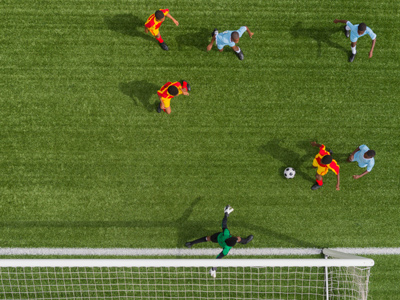An international football pitch is 105m x 68m.

# Level 3-4 Shapes - Perimeter and Area

As part of your work on shapes in KS3 Maths, you will need to know about perimeter and area. The perimeter is the total length of a shape's sides. The area is the size of a shape's surface.

There are formulae for working out perimeters and areas. Taking a square as a simple example, its perimeter is its length x 4 and its area is its length x its width. The area of a three-dimensional shape is worked out by adding the area of each of its faces together. So, for a cube, work out the area of one of its square faces and then multiply the answer by 6.

This quiz is all about the perimeter and area of 2D shapes; mainly shapes such as squares and rectangles. This will give you some easy practise in calculating perimeters and areas. You might then like to try our more advanced quiz called 'Level 5-6 Perimeter and Area 02'.

1.
If a square has sides that are 13 cm long, what is its area?
26 cm2
52 cm2
105 cm2
169 cm2
13cm x 13cm = 169cm2
2.
To calculate the area of a rectangle it is necessary to multiply the length by the width; how is this formula usually represented?
A = l x w
e = mc2
L = a/w
W = a/l
Area = length x width
3.
Which of the following shapes would you not be asked to find the perimeter or area of?
Rectangle
Sphere
Trapezium
Triangle
A sphere is a 3 dimensional shape. Remember that perimeter and area are only associated with 2 dimensional shapes
4.
What is the area of a rectangle with a length of 9 cm and a width of 7 cm?
49 cm2
54 cm2
63 cm2
77 cm2
9 x 7 = 63
5.
A rectangle has a length that is twice its width. If the length is 18 m, what is its perimeter?
36 m
48 m
54 m
72 m
The width of the rectangle must be 9 m
6.
What is the perimeter of a rectangle with a length of 28 cm and a width of 16 cm
42 cm
64 cm
74 cm
88 cm
(28 x 2) + (16 x 2) = 56 + 32 = 88
7.
What is the area of a square that has a length of 15 cm and a width of 16 cm?
225 cm2
256 cm2
240 cm2
Not a valid question
If the sides are different sizes then it cannot be a square!
8.
Which of the following is not a valid measurement for an area?
102 m2
15 m
18 mm2
52 cm2
Area is always spoken of in terms of 'square' units i.e. 18 square millimetres or 52 square centimetres. In the answers above, 15 m is only a length
9.
A rectangle is 2 metres long and 88 centimetres wide; what is its area?
0.176 metres2
1.76 metres2
3.52 metres2
176 metres2
Don't mix up the metres and centimetres mentioned in the question
10.
An international football pitch is 105 m x 68 m. What is the area of one half of the pitch?
3,570 metres2
4,624 metres2
7,140 metres2
11,025 metres2
105m x 68m = 7,140 metres2 so half the pitch's area is 7,140 metres2 ÷ 2 = 3,570 metres2